# David Silver 强化学习Lecture5：Model-Free Control

David Silver强化学习系列博客的内容整理自David Silver 强化学习的PPT和知乎叶强强化学习专栏。

# 1 Introduction

第四章节的内容讲述了agent在不依赖模型的情况下如何进行预测，也就是求解在给定策略下的状态价值或行为价值函数。本章节则主要讲解在不基于模型的条件下如何通过agent的学习优化价值函数，同时改善自身行为的策略以最大化获得累积奖励的过程，这一过程也称作不基于模型的控制。
生活中有很多关于优化控制的问题，比如控制一个大厦内的多个电梯使得效率最高；机器人足球世界杯上控制机器人球员等等。所有的这些问题要么我们对其环境动力学的特点无法掌握，但是我们可以去经历、去尝试构建理解环境的模型；要么虽然问题的环境动力学特征是已知的，但由问题的规模太大以至于计算机根据一般算法无法高效的求解，除非使用采样的办法。无论问题是属于两种情况中的哪一个，不基于模型的控制都能较好的解决。

## On and Off-Policy Learning

根据优化控制过程中是否利用已有或他人的经验策略来改进我们自身的控制策略，我们可以将这种优化控制分为两类：

# 2 On-Policy Monte-Carlo Control

## 2.1 Generalised Policy Iteration (Refresher)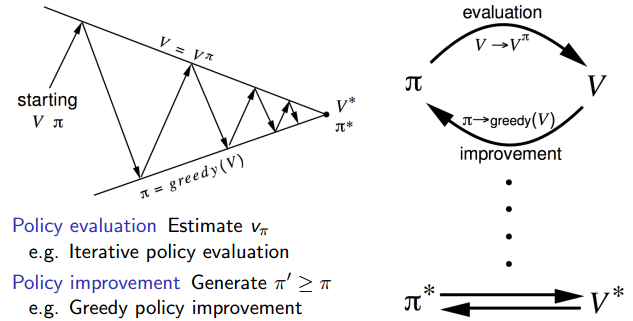通用策略迭代的核心是在两个交替的过程之间进行策略优化。一个过程是策略评估，另一个是改善策略。如上图的三角形区域所示，从一个策略$\pi$$π$和一个价值函数$V$$V$开始，每一次箭头向上代表着利用当前策略进行价值函数的更新，每一次箭头向下代表着根据更新的价值函数贪婪地选择新的策略，说它是贪婪的，是因为每次都采取转移到可能的、状态函数最高的新状态的行为。最终将收敛至最优策略和最优价值函数。
当使用动态规划算法来改善策略是需要知道某一状态的所有后续状态及状态间转移概率：${\pi }^{\prime }\left(s\right)=argma{x}_{a\in A}{R}_{s}^{a}+{P}_{s{s}^{\prime }}^{a}V\left({s}^{\prime }\right)$$\pi'(s)=argmax_{a\in A}R^a_s+P^a_{ss'}V(s')$

### 2.1.1 Generalised Policy Iteration With Monte-Carlo Evaluation

动态规划的方法是否适用于模型未知的蒙特卡洛学习呢？答案是否定的。我们无法通过分析、比较基于状态的价值来改善贪婪策略，因为不知道上述公式中当前状态的所有后续状态，以及他们的状态转移概率，进而无法确定在当前状态下采取怎样的行为更合适。
这不难理解，拿第二章节提到的学生马尔科夫决策过程的例子来说，假如需要在贪婪策略下确定学生处在第三节课时的价值，你需要比较学生在第三节课后所能采取的全部两个行为“Study”和“Pub”后状态的价值。选择“Study”比较简单，在获得一个价值为10的即时奖励后进入价值恒为 0的“Sleep”状态，此时得到在“第三节课”后选择“Study”的价值为 +10；而选择“Pub”时，计算就没那么简单了，因为在“Pub”过后，学生自己并不确定将回到哪个状态，因此无法直接用某一个状态的价值来计算“Pub”行为的价值。环境按照一定的概率（分别为 0.2, 0.4, 0.4）把学生重新分配至“第一节课”、“第二节课”或“第三节课”。也只有再知道这三个概率值后，我们才能根据后续这三个状态的价值计算得到“Pub”行为的价值为 +9.4，根据贪婪策略，学生在“第三节课”的价值为 +10。在基于采样的强化学习时，我们无法事先知道这些状态之间在不同行为下的转移概率，因而无法基于状态价值来改善我们的贪婪策略。

### 2.1.2 Model-Free Policy Iteration Using Action-Value Function

生活中也是如此，有时候一个人给自己制定了一个价值很高的目标，却发现不知采取如何的行为来达到这个目标。与其花时间比较目标与现实的差距，倒不如立足于当下，在所有可用的行为中选择一个最高价值的行为，如此来接近目标。
因此，解决这一问题的方法是，使用行为价值函数$Q\left(s,a\right)$$Q(s,a)$代替状态价值函数：
${\pi }^{\prime }\left(s\right)=argma{x}_{a\in A}Q\left(s,a\right)$$\pi'(s)=argmax_{a\in A}Q(s,a)$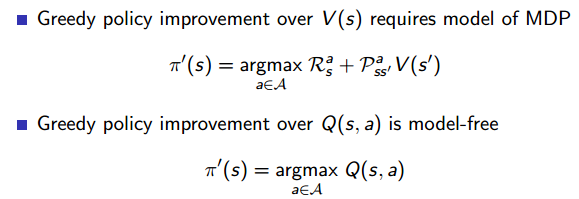这样做的目的是可以改善策略而不用知道整个模型，只需要知道在某个状态下采取什么什么样的行为使得行为价值最大即可。具体是这样：我们从一个初始的$Q$$Q$和策略$\pi$$\pi$开始，先根据这个策略更新每一个状态行为对的$q$$q$值，$s$$s$随后基于更新的$Q$$Q$确定改善的贪婪算法。
即使这样，至少还存在一个问题，即当我们每次都使用贪婪算法来改善策略的时候，将很有可能由于没有足够的采样经验而导致产生一个并不是最优的策略，我们需要不时的尝试一些新的行为，这就是探索（Exploration），使用一个示例来解释：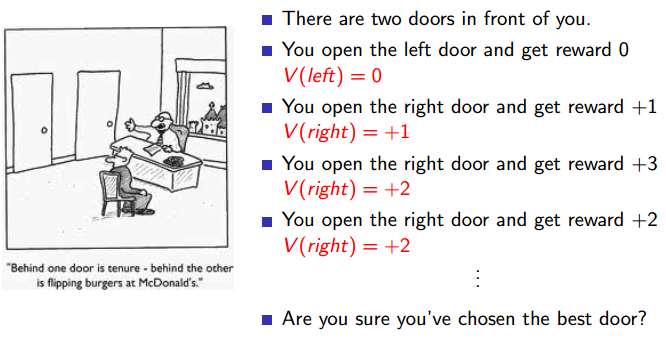如图：在你面前有两扇门，考虑如下的行为、奖励并使用贪婪算法改善策略：
你打开左侧门得到即时奖励为０：$V\left(left\right)=0$$V(left) = 0$
你打开右侧门得到即时奖励１：$V\left(right\right)=+1$$V(right) = +1$
在使用贪婪算法时，接下来你将会继续打开右侧的门，而不会尝试打开左侧门
你打开右侧门得到即时奖励＋３：$V\left(right\right)=\left(1+3\right)/2=+2$$V(right) =(1+3)/2= +2$
你打开右侧门得到即时奖励＋2：$V\left(right\right)=\left(1+3+2\right)/3=+2$$V(right) =(1+3+2)/3= +2$
这种情况下，打开右侧门是否就一定是最好的选择呢？答案显而易见是否定的。因此完全使用贪婪算法改善策略通常不能得到最优策略。

## 2.2 Exploration

### 2.2.1 ϵ$ϵ$$\epsilon$-Greedy Exploration

再举一个例子。
假设你刚搬到一个街区，街上有两家餐馆，你决定去两家都尝试一下并给自己的就餐体验打个分，分值在 0-10 分之间。你先体验了第一家，觉得一般，给了 5 分；过了几天又去了第二家，觉得不错，给了 8 分。此时，如果你选择贪婪策略，每次只去评分高的餐馆就餐，那么下一次你将继续选择去第二家餐馆。假设这次体验差了点，给了 6 分。经过了三次体验后，你对第一家餐馆的评分为 5 分，第二家的评分平均下来是 7 分。之后你仍然选择贪婪策略，下一次体验还去了第二家，假设体验为 7 分，那么经过这 4 次体验之后，你能确认对你来说第二家餐馆就一定比第一家好吗？答案是否定的，原因在于你只尝试了一次去第一家就餐，仅靠这一次的体验是不可靠的。贪婪策略并不意味着你今后就一定无法选择第一家就餐，当你每次依据贪婪算法在第二家餐馆就餐时，如果体验分降低导致平均分低于第一家的评分 5 分，那么下一次你将选择去第一家餐馆。不过如果你对第二家餐馆的平均体验分一直在第一家之上，那么依据贪婪策略将无法再去第一家参观体验了，也许你第一次去第一家餐馆就餐时恰好碰到他们刚开张管理还不完善的情况，而现在已经做得很好了。贪婪策略将使你错失第一家餐馆的许多美味了。采取贪婪策略还有一个问题，就是如果这条街上新开了一家餐馆，如果你对没有去过的餐馆评分为 0 的话，你将永远不会去尝试这家餐馆。
贪婪策略产生问题的根源是无法保证持续的探索，为了解决这一问题，我们需要引入一个随机机制，以一定的概率选择当前最好的策略，同时给其它可能的行为一定的几率，这就是$ϵ$$\epsilon$-贪婪探索。
$ϵ$$\epsilon$-贪婪探索的目标使得某一状态下所有可能的行为都有一定非零几率被选中执行，也就保证了持续的探索，$1-ϵ$$1-\epsilon$的概率下选择当前认为最好的行为，而$ϵ$$\epsilon$的概率在所有可能的$m$$m$个行为中选择（也包括那个当前最好的行为）。数学表达式如下：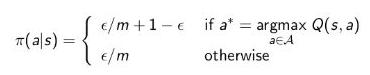有一个定理：使用$ϵ$$\epsilon$-贪婪探索策略，对于任意一个给定的策略$\pi$$\pi$，我们在评估这个策略的同时也总在改善它。

## 2.3 Monte-Carlo Control

### 2.3.1 Monte-Carlo Policy Iteration

如此，我们最终可以看到蒙特卡洛控制的全貌：使用$Ｑ$$Ｑ$函数进行策略评估，使用$ϵ$$\epsilon$-贪婪探索来改善策略。该方法最终可以收敛至最优策略。如下图所示：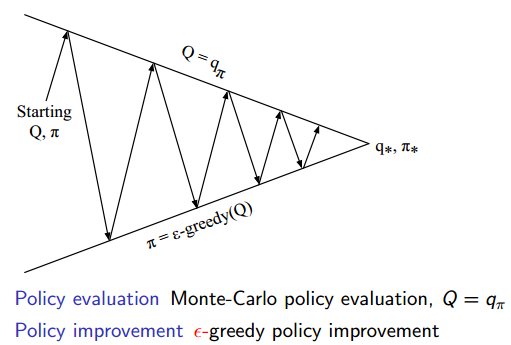图中每一个向上或向下的箭头都对应着多个Episode。也就是说我们一般在经历了多个Episode之后才进行依次Ｑ函数更新或策略改善。

### 2.3.2 GLIE

但是实际上我们也可以在每经历一个Episode之后就更新Ｑ函数或改善策略。但不管使用那种方式，在$ϵ$$\epsilon$-贪婪探索算下我们始终只能得到基于某一策略下的近似Ｑ函数，且该算法没有一个终止条件，因为它一直在进行探索。因此我们必须关注以下两个方面：一方面我们不想丢掉任何更好信息和状态，想继续探索一些随机行为，保证没有丢掉更好的策略；另一方面随着我们策略的改善我们最终希望能终止于某一个最优策略，因为一个最优策略不应该包括一些随机行为。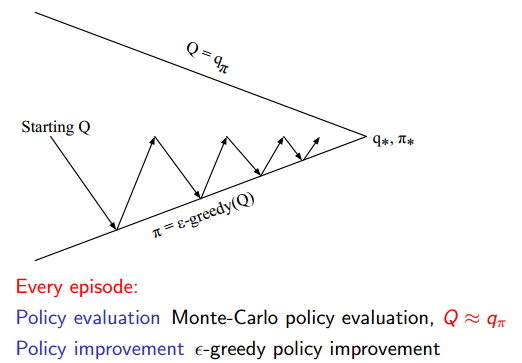为此引入了另一个理论概念：GLIE。直白的说，在有限的时间内进行无限可能的探索。它包含两层意思:

如果在使用 $ϵ$$\epsilon$-贪婪策略时，能令$ϵ$$\epsilon$随采样次数的无限增加而趋向于 0 就符合 GLIE。例如我们取$ϵ=1/k$$\epsilon = 1/k$$k$$k$为探索的Episode数目），那么该$ϵ$$\epsilon$贪婪蒙特卡洛控制就具备GLIE特性。基于 GLIE 的蒙特卡洛控制流程如下：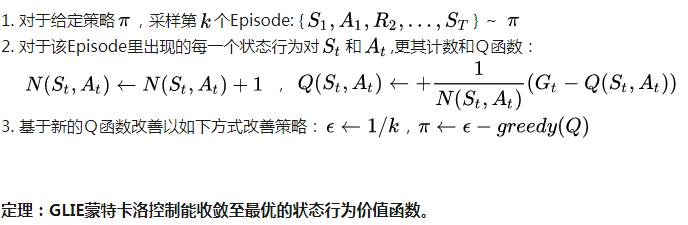# 3 On-Policy Temporal-Difference Learning

## 3.1 MC vs. TD Control

TD相比MC有很多优点：低方差，可以在线实时学习，可以学习不完整Episode等。在控制问题上使用 TD 学习同样具备上述的一些优点。这就是Sarsa和Sarsa($\lambda$$\lambda$)算法。

## 3.2 Sarsa

### Updating Action-Value Functions with Sarsa

SARSA的名称来源于下图所示的序列描述：针对一个状态$S$$S$，agent通过行为策略产生一个特定的行为$A$$A$，执行该行为进而产生一个状态行为对$\left(S,A\right)$$(S,A)$，与环境交互，环境收到agent的行为后会告诉agent即时奖励$R$$R$以及后续进入的状态${S}^{\prime }$$S'$；接下来agent再次遵循现有的行为策略产生一个新行为${A}^{\prime }$$A'$，agent此时并不执行该行为，而是根据当前的行为价值函数得到后一个状态行为对$\left({S}^{\prime },{A}^{\prime }\right)$$(S',A')$的价值$Q$$Q$，利用这个$Q$$Q$值和前面的即时奖励$R$$R$更新前一个状态行为对$\left(S,A\right)$$(S,A)$的价值。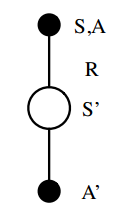与MC算法不同的是，Sarsa算法在单个状态序列内的每一个时间步，在状态S下采取一个行为A到达状态S’后都要更新状态行为对 (S,A) 的价值 Q(S,A)。这一过程同样使用 ϵ-贪婪策略进行策略迭代：
$Q\left(S,A\right)←Q\left(S,A\right)+\alpha \left(R+\gamma Q\left({S}^{\prime },{A}^{\prime }\right)-Q\left(S,A\right)\right)$$Q(S,A) \leftarrow Q(S,A)+\alpha(R+\gamma Q(S',A')-Q(S,A))$
在 Sarsa 算法中，$Q\left(S,A\right)$$Q(S,A)$ 的值使用一张大表来存储的，这不是很适合解决规模很大的问题；对于每一个状态序列Episode，在$S$$S$状态时采取的行为$A$$A$是基于当前行为策略的，同时该行为也是实际Episode发生的行为，在更新状态行为对$\left(S,A\right)$$(S,A)$的价值的循环里，agent并不实际执行在${S}^{\prime }$$S'$下的${A}^{\prime }$$A'$行为，而是将行为${A}^{\prime }$$A'$留到下一个循环执行。
在更新行为价值时，参数$\alpha$$α$是学习速率参数，$\gamma$$γ$是衰减因子。当行为策略满足前文所述的GLIE 特性，同时学习速率参数$\alpha$$α$满足：$\sum _{t=1}^{\mathrm{\infty }}{\alpha }_{t}=\mathrm{\infty },且\sum _{t=1}^{\mathrm{\infty }}{\alpha }_{t}^{2}<\mathrm{\infty }$$\sum\limits_{t=1}^{\infty}\alpha_t=\infty,且\sum\limits_{t=1}^{\infty}\alpha_t^2<\infty$时，Sarsa算法将收敛至最优策略和最优价值函数。

## 3.3 Example：Sarsa on the Windy Gridworld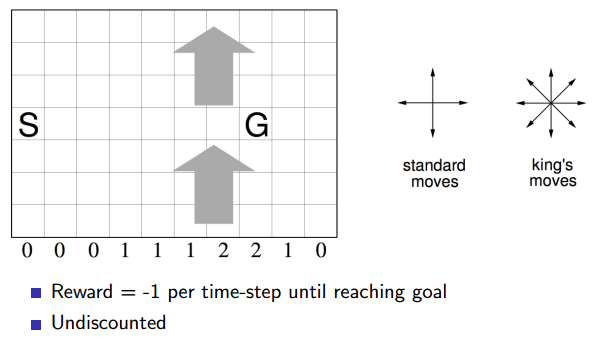如图所示，使用一个经典环境有风格子世界来解释 Sarsa 算法的学习过程。环境是一个10×7的长方形格子世界，标记有一个起始位置 S 和一个终止目标位置 G，格子下方的数字表示对应的列中一定强度的风。当agent进入该列的某个格子时，会按图中箭头所示的方向自动移动数字表示的格数，借此来模拟世界中风的作用。同样格子世界是有边界的，agent任意时刻只能处在世界内部的一个格子中。agent并不清楚这个世界的构造以及有风，也就是说它不知道格子是长方形的，也不知道边界在哪里，也不知道自己在里面移动移步后下一个格子与之前格子的相对位置关系，当然它也不清楚起始位置、终止目标的具体位置。但是agent会记住曾经经过的格子，下次在进入这个格子时，它能准确的辨认出这个格子曾经什么时候来过。格子可以执行的行为是朝上、下、左、右移动一步。现在要求解的问题是agent应该遵循怎样的策略才能尽快的从起始位置到达目标位置。
首先将这个问题用强化学习的语言再描述一遍。这是一个不基于模型的控制问题，也就是要在不掌握马尔科夫决策过程的情况下寻找最优策略。环境世界中每一个格子可以用水平和垂直坐标来描述，如此构成拥有 70 个状态的状态空间 S。行为空间 A 具有四个基本行为。环境的的动力学特征不被agent掌握，但agent每执行一个行为，会进入一个新的状态，该状态由环境告知agent，但环境不会直接告诉agent该状态的坐标位置。即时奖励是根据任务目标来设定，现要求尽快从起始位置移动到目标位置，我们可以设定每移动一步只要不是进入目标位置都给予一个 -1 的惩罚，直至进入目标位置后获得奖励 0 同时永久停留在该位置。
agent通过学习发现下面的行为序列（共15步）能够得到最大程度的奖励: -14
右、右、右、右、右、右、右、右、右、下、下、下、下、左、左
agent找到该最优策略的进度以及最优策略下agent从起始状态到目标状态的行为轨迹如图：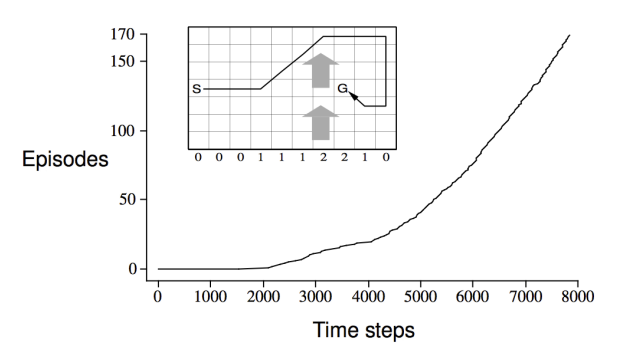在个体找到这个最优行为序列的早期，由于个体对环境一无所知，SARSA算法需要尝试许多不同的行为，因此在一开始的2000多步里，个体只能完成少数几个完整的Episode，但随着个体找到一条链接起点到终点的路径，其快速优化策略的能力就显现的很明显了，因为它不需要走完一个Episode才能更新行为价值，而是每走一步就根据下一个状态能够得到的最好价值来更新当前状态的价值。

## 3.4 Sarsa(λ)

### 3.4.1 n-Step Sarsa

根据前面的n-步收获，类似的可以引出一个 n-步 Sarsa 的概念。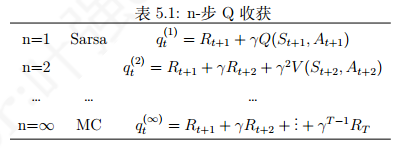这里的${q}_{t}$$q_t$对应的是一个状态行为对$<{s}_{t},{a}_{t}>$$$，表示的是在某个状态下采取某个行为的价值大小。如果$n=1$$n = 1$，则表示状态行为对$<{s}_{t},{a}_{t}>$$$$Q$$Q$价值可以用两部分表示，一部分是离开状态${s}_{t}$$s_t$得到的即时奖励${R}_{t+1}$$R_{t+1}$，即时奖励只与状态有关，与该状态下采取的行为无关；另一部分是新状态行为对$<{s}_{t+1},{a}_{t+1}>$$$$Q$$Q$价值：环境给了个体一个新状态${s}_{t+1}$$s_{t+1}$，观察在${s}_{t+1}$$s_{t+1}$状态时基于当前策略得到的行为${a}_{t+1}$$a_{t+1}$时的$Q\left({s}_{t+1},{a}_{t+1}\right)$$Q(s_{t+1}, a_{t+1})$，后续的$Q$$Q$价值考虑衰减系数。当$n=2$$n = 2$时，就向前用$2$$2$步的即时奖励，然后再用新状态的$Q$$Q$价值代替；如果$n=\mathrm{\infty }$$n = \infty$，则表示一直用即时奖励计算$Q$$Q$值，直至Episode结束，agent进入终止状态，获得终止状态的即时奖励。
定义n-步Q收获（Q-return）：
${q}_{t}^{\left(n\right)}={R}_{t+1}+\gamma {R}_{t+2}+...+{\gamma }^{n-1}{R}_{t+n}+{\gamma }^{n}Q\left({S}_{t+n},{A}_{t+n}\right)$$q_t^{(n)}=R_{t+1}+\gamma R_{t+2}+...+\gamma^{n-1}R_{t+n}+\gamma ^nQ(S_{t+n},A_{t+n})$
上式和之前的n-步G收获很相似，这里的n-步Q收获，Q是包含行为的，也就是在当前策略下基于某一状态产生的行为。
有了如上定义，可以把n-步Sarsa用n-步Q收获来表示，如下式：
$Q\left({S}_{t},{A}_{t}\right)←Q\left({S}_{t},{A}_{t}\right)+\alpha \left[{q}_{t}^{\left(n\right)}-Q\left({S}_{t},{A}_{t}\right)\right]$$Q(S_t,A_t)\leftarrow Q(S_t,A_t)+\alpha[q^{(n)}_t-Q(S_t,A_t)]$
类似于 TD(λ), 可以给 n-步 Q 收获中的每一步收获分配一个权重，并按权重对每一步 Q 收获求和，那么将得到 ${q}^{\lambda }$$q^λ$ 收获，它结合了所有 n-步 Q 收获：
${q}_{t}^{\lambda }=\left(1-\lambda \right)\sum _{n=1}^{\mathrm{\infty }}{\lambda }^{n-1}{q}_{t}^{\left(n\right)}$$q_t^{\lambda}=(1-\lambda)\sum\limits_{n=1}^{\infty}\lambda^{n-1}q^{(n)}_t$

### 3.4.2 Forward View Sarsa(λ)

如果用某一状态的${q}^{\lambda }$$q^\lambda$收获来更新状态行为对的$Q$$Q$值，那么可以表示称如下的形式：
$Q\left({S}_{t},{A}_{t}\right)←Q\left({S}_{t},{A}_{t}\right)+\alpha \left({q}_{t}^{\left(\lambda \right)}-Q\left({S}_{t},{A}_{t}\right)\right)$$Q(S_t, A_t) \leftarrow Q(S_t, A_t) + α (q_t^{(λ)} − Q(S_t, A_t))$
这是Sarsa(λ) 的前向认识，使用它更新 Q 价值需要遍历完整的状态序列。

### 3.4.3 Backward View Sarsa(λ)

与TD(λ)的反向认识一样，引入效用追踪（Eligibility Trace）概念，不同的是这次的E值针对的不是一个状态，而是一个状态行为对：
${E}_{0}\left(s,a\right)=0$$E_0(s, a) = 0$
${E}_{t}\left(s,a\right)=\gamma \lambda {E}_{t-1}\left(s,a\right)+1\left({S}_{t}=s,{A}_{t}=a\right)$$E_t(s, a) = γλE_{t−1}(s, a) + 1(S_t = s, A_t = a)$
它体现的是一个结果与某一个状态行为对的因果关系，与得到结果最近的状态行为对，以及那些在此之前频繁发生的状态行为对对得到这个结果的影响最大。
下式是引入ET概念的$SARSA\left(\lambda \right)$$SARSA(\lambda)$之后的Q值更新描述：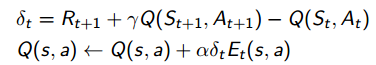引入ET概念，同时使用$SARSA\left(\lambda \right)$$SARSA(\lambda)$将可以更有效的在线学习，因为不必要学习完整的Episode，数据用完即可丢弃。ET通常也是更多应用在在线学习算法中(online algorithm)。

### 3.4.4 Sarsa(λ) Gridworld Example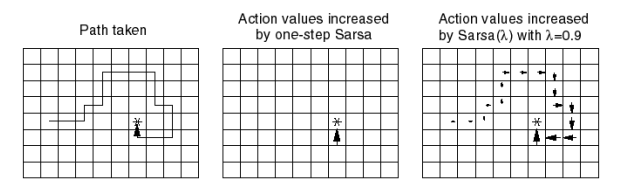接下来用格子世界的例子具体解释了 Sarsa 和 Sarsa(λ) 算法的区别：假设图最左侧描述的路线是agent采取两种算法中的一个得到的一个完整状态序列的路径。为了下文更方便描述、解释两个算法之间的区别，先做几个合理的小约定：
1) 认定每一步的即时奖励为 0，直到终点处即时奖励为 1；
2) 根据算法，除了终点以外的任何状态行为对的 Q 值可以在初始时设为任意的，但我们设定所有的 Q 值均为 0；
3) 该路线是agent第一次找到终点的路线。
Sarsa（0） 算法：
由于是现时策略学习，一开始个体对环境一无所知，即所有的 $Q$$Q$ 值均为 0，它将随机选取移步行为。在到达终点前的每一个位置 $S$$S$，agent依据当前策略，产生一个移步行为，执行该行为，环境会将其放置到一个新位置 $S\prime$$S′$，同时给以即时奖励 $0$$0$，在这个新位置上，根据当前的策略它会产生新位置下的一个行为，agent不执行该行为，仅仅在表中查找新状态下新行为的$Q$$Q$值，由于 Q = 0，依据更新公式，它将把刚才离开的位置以及对应的行为的状态行为对价值$Q\left(S,A\right)$$Q(S,A)$更新为 0。如此直到个体最到达终点位置 ${S}_{G}$$S_G$，它获得一个即时奖励 $1$$1$，此时agent会依据公式更新其到达终点位置前所在那个位置（暂用 ${S}_{H}$$S_H$ 表示，也就是终点位置下方，向上的箭头所在的位置）时采取向上移步的那个状态行为对价值 $Q\left({S}_{H},{A}_{up}\right)$$Q(S_H, A_{up})$，它将不再是 0，这是agent在这个状态序列中唯一一次用非 0 数值来更新 $Q$$Q$ 值。这样完成一个Episode，此时agent已经并只进行了一次有意义的行为价值函数的更新；同时依据新的价值函数产生了新的策略。这个策略绝大多数与之前的相同，只是当agent处在特殊位置 ${S}_{H}$$S_H$ 时将会有一个近乎确定的向上的行为。这里请不要误认为 Sarsa 算法只在经历一个完整的状态序列之后才更新，在这个例子中，由于我们的设定，它每走一步都会更新，只是多数时候更新的数据和原来一样罢了。
此时如果要求agent继续学习，则环境将其放入起点。个体的第二次寻路过程一开始与首次一样都是盲目随机的，直到其进入终点位置下方的位置 ${S}_{H}$$S_H$，在这个位置，agent更新的策略将使其有非常大的几率选择向上的行为直接进入终点位置 ${S}_{G}$$S_G$
同样，经过第二次的寻路，agent了解到到达终点下方的位置 ${S}_{H}$$S_H$ 价值比较大，因为在这个位置直接采取向上移步的行为就可以拿到到达终点的即时奖励。因此它会将那些通过移动一步就可以到达 ${S}_{H}$$S_H$ 位置的其它位置以及相应的到达该位置位置所要采取的行为对所对应的价值进行提升。如此反复，如果采用贪婪策略更新，agent最终将得到一条到达终点的路径，不过这条路径的倒数第二步永远是在终点位置的下方。如果采用 $ϵ$$\epsilon$-贪婪策略更新，那么个体还会尝试到终点位置的左上右等其它方向的相邻位置价值也比较大，此时个体每次完成的路径可能都不一样。通过重复多次搜索，这种 $Q$$Q$ 值的实质有意义的更新将覆盖越来越多的状态行为对，agent在早期采取的随机行为的步数将越来越少，直至最终实质性的更新覆盖到起始位置。此时agent将能直接给出一条确定的从起点到终点的路径。
Sarsa(λ) 算法：
该算法同时还针对每一次状态序列维护一个关于状态行为对 $\left(S,A\right)$$(S, A)$$E$$E$ 表，初始时 $E$$E$ 表值均为 0。当agent首次在起点 ${S}_{0}$$S_0$ 决定移动一步 ${A}_{0}$$A_0$(假设向右) 时，它被环境告知新位置为 ${S}_{1}$$S_1$，此时发生如下事情：
首先，agent会做一个标记，使 $E\left({S}_{0},{A}_{0}\right)$$E(S_0, A_0)$ 的值增加 1，表明agent刚刚经历过这个事件 $\left({S}_{0},{A}_{0}\right)$$(S_0, A_0)$
其次，它要估计这个事件的对于解决整个问题的价值，也就是估计TD误差，此时依据公式结果为 0，说明agent认为在起点处向右走没什么价值，这个“没有什么价值”有两层含义：不仅说明在 ${S}_{0}$$S_0$ 处往右目前对解决问题没有积极帮助，同时表明agent认为所有能够到达 ${S}_{0}$$S_0$ 状态的状态行为对的价值没有任何积极或消极的变化。
随后，agent将要更新该状态序列中所有已经经历的 $Q\left(S,A\right)$$Q(S, A)$ 值，由于存在 $E$$E$ 值，那些在 $\left({S}_{0},{A}_{0}\right)$$(S_0, A_0)$ 之前近期发生或频繁发生的 $\left(S,A\right)$$(S, A)$$Q$$Q$ 值将改变得比其它 $Q$$Q$ 值明显些，此外agent还要更新其 $E$$E$ 值，以备下次使用。对于刚从起点出发的agent，这次更新没有使得任何 $Q$$Q$ 值发生变化，仅仅在 $E\left({S}_{0},{A}_{0}\right)$$E(S_0, A_0)$ 处有了一个实质的变化。随后的过程类似，agent有意义的发现就是对路径有一个记忆，体现在 $E$$E$ 里，具体的 $Q$$Q$ 值没发生变化。这一情况直到agent到达终点位置时发生改变。此时agent得到了一个即时奖励 1，它会发现这一次变化（从 ${S}_{H}$$S_H$ 采取向上行为 ${A}_{up}$$A_{up}$ 到达 ${S}_{G}$$S_G$）价值明显，它会计算这个 TD误差为 1，同时告诉整个经历过程中所有$\left(S,A\right)$$(S, A)$，根据其与 $\left({S}_{H},{A}_{up}\right)$$(S_H, A_{up})$ 的密切关系更新这些状态行为对的价值$Q$$Q$，agent在这个状态序列中经历的所有状态行为对的$Q$$Q$值都将得到一个非 0 的更新，但是那些在个体到达 ${S}_{H}$$S_H$ 之前就近发生以及频繁发生的状态行为对的价值提升得更加明显。
在图示的例子中没有显示某一状态行为频发的情况，如果agent在寻路的过程中绕过一些弯，多次到达同一个位置，并在该位置采取的相同的动作，最终agent到达终止状态时，就产生了多次发生的 $\left(S,A\right)$$(S, A)$，这时的 $\left(S,A\right)$$(S, A)$ 的价值也会得到较多提升。也就是说，agent每得到一个即时奖励，同时会对所有历史事件的价值进行依次更新，当然那些与该事件关系紧密的事件价值改变的较为明显。这里的事件指的就是状态行为对。在同一状态采取不同行为是不同的事件。
当agent重新从起点第二次出发时，它会发现起点处向右走的价值不再是 0。如果采用贪婪策略更新，agent将根据上次经验得到的新策略直接选择右走，并且一直按照原路找到终点。如果采用 ϵ-贪婪策略更新，那么agent还会尝试新的路线。由于为了解释方便，做了一些约定，这会导致问题并不要求agent找到最短一条路径，如果需要找最短路径，需要在每一次状态转移时给agent一个负的奖励。
Sarsa(λ)算法里在状态每发生一次变化后都对整个状态空间和行为空间的Q和E值进行更新，而事实上在每一个Episode里，只有agent经历过的状态行为对的E才可能不为0，为什么不仅仅对该Episode涉及到的状态行为对进行更新呢？理论上是可以仅对Episode里涉及的状态行为对的E和Q进行更新的，不过这要额外维护一个表，而往这个额外的表里添加新的状态行为对的E和Q值比更新总的状态行为空间要麻烦，特别是在早期agent没有一个较好的策略的时候需要花费很长很长时间才能找到终点位置，这在一定程度上反而没有更新状态空间省时。不过随着学习深入、策略得到优化，此表的规模会变小。

# 4 Off-Policy Learning

现时策略学习的特点就是产生实际行为的策略与更新价值 (评价) 所使用的策略是同一个策略，而借鉴策略学习 (oﬀ-policy learning) 中产生指导自身行为的策略 $µ\left(a|s\right)$$µ(a|s)$ 与评价策略 $\pi \left(a|s\right)$$π(a|s)$是不同的策略，具体地说，agent通过策略$µ\left(a|s\right)$$µ(a|s)$ 生成行为与环境发生实际交互，但是在更新这个状态行为对的价值时使用的是目标策略$\pi \left(a|s\right)$$π(a|s)$。目标策略$\pi \left(a|s\right)$$π(a|s)$多数是已经具备一定能力的

## 4.1 Importance Sampling for Off-Policy TD

借鉴学习TD学习任务就是使用TD方法在目标策略 $\pi \left(a|s\right)$$π(a|s)$的基础上更新行为价值，进而优化行为策略：
$V\left({S}_{t}\right)←V\left({S}_{t}\right)+\alpha \left[\frac{\pi \left({A}_{t}|{S}_{t}\right)}{µ\left({A}_{t}|{S}_{t}\right)}\left({R}_{t+1}+\gamma V\left({S}_{t+1}\right)\right)-V\left({S}_{t}\right)\right]$$V(S_t)\leftarrow V(S_t)+\alpha[\frac{\pi(A_t|S_t)}{µ(A_t|S_t)}(R_{t+1}+\gamma V(S_{t+1}))-V(S_t)]$
对于上式，我们可以这样理解：agent处在状态 ${S}_{t}$$S_t$ 中，基于行为策略$µ$$µ$产生了一个行为${A}_{t}$$A_t$，执行该行为后进入新的状态 ${S}_{t+1}$$S_{t+1}$，借鉴策略学习要做的事情就是，比较借鉴策略和行为策略在状态 ${S}_{t}$$S_t$ 下产生同样的行为 ${A}_{t}$$A_t$ 的概率的比值，如果这个比值接近 $1$$1$，说明两个策略在状态 ${S}_{t}$$S_t$ 下采取的行为 ${A}_{t}$$A_t$ 的概率差不多，此次对于状态 ${S}_{t}$$S_t$ 价值的更新同时得到两个策略的支持。如果这一概率比值很小，则表明借鉴策略 $\pi$$π$ 在状态 ${S}_{t}$$S_t$ 下选择 ${A}_{t}$$A_t$ 的机会要小一些，此时为了从借鉴策略学习，我们认为这一步状态价值的更新不是很符合借鉴策略，因而在更新时打些折扣。类似的，如果这个概率比值大于 1，说明按照借鉴策略，选择行为 ${A}_{t}$$A_t$ 的几率要大于当前行为策略产生 ${A}_{t}$$A_t$的概率，此时应该对该状态的价值更新就可以大胆些。

### Q-Learning

借鉴策略 TD学习中一个典型的行为策略$µ$$µ$是基于行为价值函数$Q\left(s,a\right)$$Q(s, a)$$ϵ$$ϵ$-贪婪策略，借鉴策略$\pi$$π$则是基于$Q\left(s,a\right)$$Q(s, a)$的完全贪婪策略，这种学习方法称为 Q 学习 (Q learning)。
Q 学习的目标是得到最优价值$Q\left(s,a\right)$$Q(s,a)$，在 Q 学习的过程中， $t$$t$ 时刻的与环境进行实际交互的行为${A}_{t}$$A_t$ 由策略 $µ$$µ$ 产生：${A}_{t}\sim µ\left(·|{S}_{t}\right)$$A_t∼ µ(·|S_t)$
其中策略 $µ$$µ$是一个 ϵ-贪婪策略。 $t+1$$t + 1$ 时刻用来更新 $Q$$Q$ 值的行为 $A{\prime }_{t+1}$$A′ _{t+1}$ 由下式产生：$A{\prime }_{t+1}\sim \pi \left(·|{S}_{t+1}\right)$$A′ _{t+1}∼ π(·|S_{t+1})$
其中策略 $\pi$$π$是一个完全贪婪策略。 $Q\left({S}_{t},{A}_{t}\right)$$Q(S_t, A_t)$ 的按下式更新：
$Q\left({S}_{t},{A}_{t}\right)←Q\left({S}_{t},{A}_{t}\right)+\alpha \left({R}_{t+1}+\gamma Q\left({S}_{t+1},A\prime \right)-Q\left({S}_{t},{A}_{t}\right)\right)$$Q(S_t, A_t) \leftarrow Q(S_t, A_t) + α(R_{t+1} + γQ(S_{t+1}, A′) − Q(S_t, A_t))$
其中，${R}_{t+1}+\gamma Q\left({S}_{t+1},A\prime \right)$$R_{t+1} + γQ(S_{t+1}, A′)$部分的 TD 目标是基于借鉴策略$\pi$$π$产生的行为${A}^{\prime }$$A’$ 得到的$Q$$Q$ 值。根据这种价值更新的方式，状态 ${S}_{t}$$S_t$ 依据 ϵ-贪婪策略得到的行为${A}_{t}$$A_t$ 的价值将朝着 ${S}_{t+1}$$S_{t+1}$ 状态下贪婪策略确定的最大行为价值的方向做一定比例的更新。这种算法能够使个体的行为策略策略 $µ$$µ$ 更加接近贪婪策略，同时保证保证个体能持续探索并经历足够丰富的新状态。并最终收敛至最优策略和最优行为价值函数。
$Q$$Q$ 学习具体的行为价值更新公式：
$Q\left({S}_{t},{A}_{t}\right)←Q\left({S}_{t},{A}_{t}\right)+\alpha \left({R}_{t+1}+\gamma \underset{{a}^{\prime }}{max}Q\left({S}_{t+1},{a}^{\prime }\right)-Q\left({S}_{t},{A}_{t}\right)\right)$$Q(S_t, A_t) \leftarrow Q(S_t, A_t) + α(R_{t+1} + γ\max\limits_{a'}Q(S_{t+1},a') − Q(S_t, A_t))$©️2019 CSDN 皮肤主题: 大白 设计师: CSDN官方博客